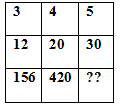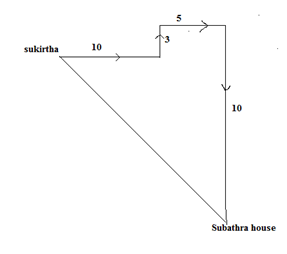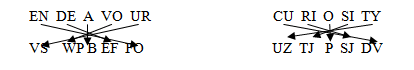# SSC CGL 2018 Practice Test Papers | Reasoning Ability (Day-16)

Dear Aspirants, Here we have given the Important SSC CGL Exam 2018 Practice Test Papers. Candidates those who are preparing for SSC CGL 2018 can practice these questions to get more confidence to Crack SSC CGL 2018 Examination.

[WpProQuiz 2893]

Click “Start Quiz” to attend these Questions and view Explanation

1) Find out the odd word/letters/number/number pair from the given alternatives.

a) Amoeba

b) Bacteria

c) Germs

d) Microbes

2) Find out the odd word/letters/number/number pair from the given alternatives.

a) Skin

b) Mouth

c) Fingers

d)   Heart

3)  Find the missing letter in the given table.a) 560

b) 680

c) 725

d) 930

(4-6). Five members M, N, O, P and Q are sitting in a straight line facing south direction. One person sits between M and Q and two persons sit between O and P. P is sitting immediate right of Q who sits at one end of the line.

4) Which of the following is true with respect to the given arrangement?

a) M sits in one end of the line

b) There are 2 persons between R and P.

c) R sits to the right of M.

d) None of the above

5) Who is sitting between M and Q?

a) O

b) R

c) P

d) Both R and P

6) Which two persons sitting at extreme ends of the line?

a) P and Q

b) M and O

c) O and Q

d) R and Q

7) Sukirtha is facing east direction. She started walking to reach her friend Subathra’s house. She walks 10 km straight and then turns left and walks for 3 km and then turns right and walks for 5 km, again a right turn and walks for 10 km. What is the shortest between Sukirtha’s starting point and subathra’s house and Subathra’s house is in which direction from her starting point?

a) 5, North-west

b) 5, South-east

c) 5, South-east

d) 5, North-west

8) In a certain coding language, ‘ENDEAVOUR’ is coded as ‘VSWPBEFFO’ how CURIOSITY is coded in that language?

a) UZTJPSJDV

b) SDFJPSJDV

c) WEFPYTEDS

d) QWEGHJUES

9) The following Equations follow certain pattern. Find the answer for the following.

6*F=J

3*I=A

2*R=??
a) M

b) T

c) J

d) O

10) If \$ means ‘added to’, # means ‘multiplied by’, % means ‘divided by’ and & means ‘difference of’ then what is the value of 7 \$ 55 % 11 # 9& 45.

a) 9

b) 7

c) -7

d) -9

Except amoeba all other are multi cellular organisms.

Except d all others are external organs.

3*4=12; 12*13=156

4*5=20; 20*21=420

5*6=30; 30*31=930

ORMPQ

ORMPQ

ORMPQ6*6(F)   = 36 = 36-26 =10= J

3*9(I)    = 27 = 27-26 =1= A

2*18(R) = 36 = 36-26 =10=J